JEE  >  Test: Comprehension Based Questions: Probability

# Test: Comprehension Based Questions: Probability

Test Description

## 16 Questions MCQ Test Maths 35 Years JEE Main & Advanced Past year Papers | Test: Comprehension Based Questions: Probability

Test: Comprehension Based Questions: Probability for JEE 2023 is part of Maths 35 Years JEE Main & Advanced Past year Papers preparation. The Test: Comprehension Based Questions: Probability questions and answers have been prepared according to the JEE exam syllabus.The Test: Comprehension Based Questions: Probability MCQs are made for JEE 2023 Exam. Find important definitions, questions, notes, meanings, examples, exercises, MCQs and online tests for Test: Comprehension Based Questions: Probability below.
Solutions of Test: Comprehension Based Questions: Probability questions in English are available as part of our Maths 35 Years JEE Main & Advanced Past year Papers for JEE & Test: Comprehension Based Questions: Probability solutions in Hindi for Maths 35 Years JEE Main & Advanced Past year Papers course. Download more important topics, notes, lectures and mock test series for JEE Exam by signing up for free. Attempt Test: Comprehension Based Questions: Probability | 16 questions in 30 minutes | Mock test for JEE preparation | Free important questions MCQ to study Maths 35 Years JEE Main & Advanced Past year Papers for JEE Exam | Download free PDF with solutions
 1 Crore+ students have signed up on EduRev. Have you?
Test: Comprehension Based Questions: Probability - Question 1

### PASSAGE - 1 There are n urns, each of these contain n + 1 balls. The ith urn contains i white balls and (n + 1 – i) red balls. Let ui be the event of selecting ith urn, i = 1, 2, 3 .........., n and w the event of getting a white ball. Q. If P(ui) ∝ i, where i = 1, 2, 3,......., n, then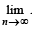P(w) =(2006 - 5M, –2)

Detailed Solution for Test: Comprehension Based Questions: Probability - Question 1

P(ui) ∝ i ⇒ P(ui)= ki, But ∑ P(ui)=1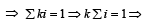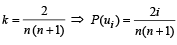By total prob. theorem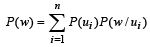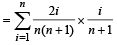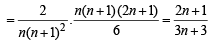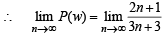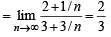Test: Comprehension Based Questions: Probability - Question 2

### PASSAGE - 1 There are n urns, each of these contain n + 1 balls. The ith urn contains i white balls and (n + 1 – i) red balls. Let ui be the event of selecting ith urn, i = 1, 2, 3 .........., n and w the event of getting a white ball. Q. If P(ui) = c, (a constant) then P(un/w) = (2006 - 5M, –2)

Detailed Solution for Test: Comprehension Based Questions: Probability - Question 2

P(ui) = c
Using Baye’s theorem,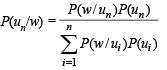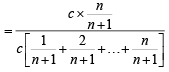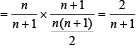Test: Comprehension Based Questions: Probability - Question 3

### PASSAGE - 1 There are n urns, each of these contain n + 1 balls. The ith urn contains i white balls and (n + 1 – i) red balls. Let ui be the event of selecting ith urn, i = 1, 2, 3 .........., n and w the event of getting a white ball. Q. Let P(ui) =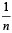, if n is even and E denotes the event of choosing even numbered urn, then the value of P(w / E) is (2006 - 5M, –2)

Detailed Solution for Test: Comprehension Based Questions: Probability - Question 3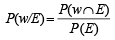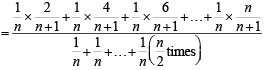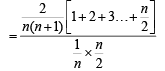(n being even)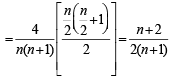Test: Comprehension Based Questions: Probability - Question 4

PASSAGE - 2
A fair die is tossed repeatedly until a six is obtained. Let X denote the number of tosses required. (2009)

Q. The probability that X = 3 equals

Detailed Solution for Test: Comprehension Based Questions: Probability - Question 4

P ( X = 3) = (probability of not a six in first chance)  × (probability of not a six in second chance)  × (probability of a six in third chance)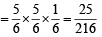Test: Comprehension Based Questions: Probability - Question 5

PASSAGE - 2
A fair die is tossed repeatedly until a six is obtained. Let X denote the number of tosses required. (2009)

Q. 5. The probability that X ≥ 3 equals

Detailed Solution for Test: Comprehension Based Questions: Probability - Question 5

P( X ≥ 3) = 1- (X< 3) = 1 -[P( X = 1) + P(X= 2)]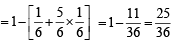Test: Comprehension Based Questions: Probability - Question 6

PASSAGE - 2
A fair die is tossed repeatedly until a six is obtained. Let X denote the number of tosses required. (2009)

Q.The conditional probability that X ≥ 6 given X > 3 equals

Detailed Solution for Test: Comprehension Based Questions: Probability - Question 6

Let us define the events A≡X≥ 6 and B ≡X>3 so that  A∩B ≡ X ≥ 6≡A

Now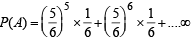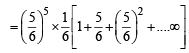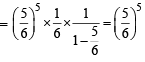and P(B)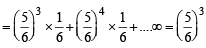∴    P(A/B) =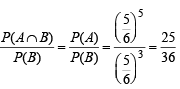Test: Comprehension Based Questions: Probability - Question 7

PASSAGE - 3
Let U1 and U2 be two urns such that U1 contains 3 white and 2 red balls, and U2 contains only 1 white ball. A fair coin is tossed. If head appears then 1 ball is drawn at random from U1 and put into U2.
However, if tail appears then 2 balls are drawn at random from U1 and put into U2 . Now 1 ball is drawn at random from U2. (2011)

Q. The probability of the drawn ball from U2 being white is

Detailed Solution for Test: Comprehension Based Questions: Probability - Question 7

P (white) = P (H ∩ white) + P ( T ∩ white) = P (H) P (white/H) + P (T) P (white/T)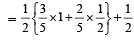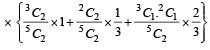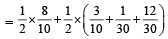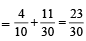Test: Comprehension Based Questions: Probability - Question 8

PASSAGE - 3
Let U1 and U2 be two urns such that U1 contains 3 white and 2 red balls, and U2 contains only 1 white ball. A fair coin is tossed. If head appears then 1 ball is drawn at random from U1 and put into U2.
However, if tail appears then 2 balls are drawn at random from U1 and put into U2 . Now 1 ball is drawn at random from U2. (2011)

Q. Given that the drawn ball from U2 is white, the probability that head appeared on the coin is

Detailed Solution for Test: Comprehension Based Questions: Probability - Question 8

P(H/white) =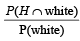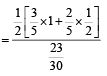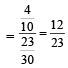Test: Comprehension Based Questions: Probability - Question 9

PASSAGE - 4
A box B1 contains 1 white ball, 3 red balls and 2 black balls. Another box B2 contains 2 white balls, 3 red balls and 4 black balls. A third box B3 contains 3 white balls, 4 red balls and 5 black balls.

Q. If 1 ball is drawn from each of the boxes B1, B2 and B3, the probability that all 3 drawn balls are of the same colour is (JEE Adv. 2013)

Detailed Solution for Test: Comprehension Based Questions: Probability - Question 9

Probability that all balls are of same colour = P (all red) + P (all white) + P (all black)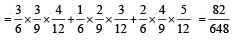Test: Comprehension Based Questions: Probability - Question 10

PASSAGE - 4
A box B1 contains 1 white ball, 3 red balls and 2 black balls. Another box B2 contains 2 white balls, 3 red balls and 4 black balls. A third box B3 contains 3 white balls, 4 red balls and 5 black balls.

Q. If 2 balls are drawn (without replacement) from a randomly selected box and one of the balls is white and the other ball is red, the probability that these 2 balls are drawn from box B2 is

Detailed Solution for Test: Comprehension Based Questions: Probability - Question 10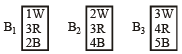Let E1, E2, E3 be the events that bag B1, B2 and B3 is selected respectively.
Let E be the event that one white and one red ball is selected.
Then by baye’s theorem,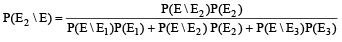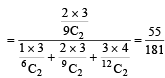Test: Comprehension Based Questions: Probability - Question 11

PASSAGE - 5
Box 1 contains three cards bearing numbers 1, 2, 3; box 2 contains five cards bearing numbers 1, 2, 3, 4, 5; and box 3 contains seven cards bearing numbers 1, 2, 3, 4, 5, 6, 7. A card is drawn from each of the boxes. Let xi be number on the card drawn from the ith box, i = 1, 2, 3. (JEE Adv. 2014)

Q. The probability that x1 + x2 + x3 is odd, is

Detailed Solution for Test: Comprehension Based Questions: Probability - Question 11

x1 + x2 + x3 will be odd If two are even and one is odd or all three are odd.

∴ Required probability = P (EEO) + P(EOE) + P(OEE) + P(OQO)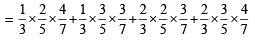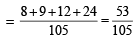Test: Comprehension Based Questions: Probability - Question 12

PASSAGE - 5
Box 1 contains three cards bearing numbers 1, 2, 3; box 2 contains five cards bearing numbers 1, 2, 3, 4, 5; and box 3 contains seven cards bearing numbers 1, 2, 3, 4, 5, 6, 7. A card is drawn from each of the boxes. Let xi be number on the card drawn from the ith box, i = 1, 2, 3. (JEE Adv. 2014)

Q. 12. The probability that x1, x2, x3 are in an arithmetic progression, is

Detailed Solution for Test: Comprehension Based Questions: Probability - Question 12

If x1, x2, x3 are in AP then 2x2 = x1 + x3

∵  LHS is even, x1 & x3 can be both even or both odd. x1 and x3 both can be even in 1 × 3 = 3 ways x1 and x3 both can be odd in 2 × 4 = 8 ways
∴ Total favourable ways = 3 + 8 = 11
Also one number from each box can be drawn in 3 × 5 × 7 ways
∴ Total ways = 105 Hence required probability =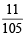*Multiple options can be correct
Test: Comprehension Based Questions: Probability - Question 13

PASSAGE - 6
Let n1 and n2 be the number of red and black balls, respectively, in box I. Let n3 and n4 be the number of red and black balls, respectively, in box II. (JEE Adv. 2015)

Q. One of the two boxes, box I and box II, was selected at random and a ball was drawn randomly out of this box. The ball was found to be red. If the probability that this red ball was drawn from box II is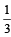, then the correct option(s) with the possible values of n1, n2, n3 and n4 is(are)

Detailed Solution for Test: Comprehension Based Questions: Probability - Question 13

Let E1 ≡ box I is selected
E2 ≡ box II is selected
E ≡ ball drawn is red

P(E2/E) =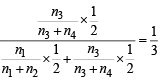or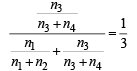On checking the options we find (a) and (b) are the correct options.

*Multiple options can be correct
Test: Comprehension Based Questions: Probability - Question 14

PASSAGE - 6
Let n1 and n2 be the number of red and black balls, respectively, in box I. Let n3 and n4 be the number of red and black balls, respectively, in box II. (JEE Adv. 2015)

Q. A ball is drawn at random from box I and transferred to box II. If the probability of drawing a red ball from box I, after this transfer, is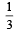, then the correct option(s) with the possible values of n1 and n2 is(are)

Detailed Solution for Test: Comprehension Based Questions: Probability - Question 14

E1 ≡ Red ball is selected from box I
E2 ≡ Black ball is selected from box I
E ≡ Second ball drawn from box I is red
∴ P(E) = P(E1) P(E/E1) + P(E2) P(E/E2)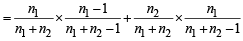On checking the options, we find (c) and (d) have the correct values.

Test: Comprehension Based Questions: Probability - Question 15

PASSAGE - 7
Football teams T1 and T2 have to play two games against each other. It is assumed that the outcomes of the two games are independent. The probabilities of T1 winning, drawing and losing a game against T2 are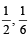andrespectively. Each team gets 3 points for a win, 1 point for a draw and 0 point for a loss in a game.
Let X and Y denote the total points scored by teams T1 and T2 respectively after two games.

Q.P (X > Y) is (JEE Adv. 2016)

Detailed Solution for Test: Comprehension Based Questions: Probability - Question 15

(X, Y) = {(6, 0), (4, 1), (3, 3), (2, 2), (4, 4), (0, 6)}
P(X > Y) = P(T1 wins 2 games or T1 win one game other is a draw)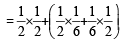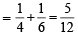Test: Comprehension Based Questions: Probability - Question 16

PASSAGE - 7
Football teams T1 and T2 have to play two games against each other. It is assumed that the outcomes of the two games are independent. The probabilities of T1 winning, drawing and losing a game against T2 areandrespectively. Each team gets 3 points for a win, 1 point for a draw and 0 point for a loss in a game.
Let X and Y denote the total points scored by teams T1 and T2 respectively after two games.

Q. P (X = Y) is (JEE Adv. 2016)

Detailed Solution for Test: Comprehension Based Questions: Probability - Question 16

(X, Y) = {(6, 0), (4, 1), (3, 3), (2, 2), (4, 4), (0, 6)}

P(X = Y) = P(T1 wins 1 game loses other game or both the games draw)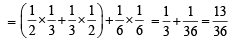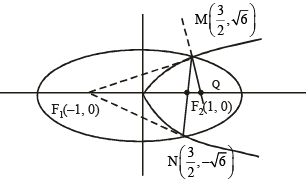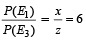## Maths 35 Years JEE Main & Advanced Past year Papers

132 docs|70 tests
 Use Code STAYHOME200 and get INR 200 additional OFF Use Coupon Code
Information about Test: Comprehension Based Questions: Probability Page
In this test you can find the Exam questions for Test: Comprehension Based Questions: Probability solved & explained in the simplest way possible. Besides giving Questions and answers for Test: Comprehension Based Questions: Probability, EduRev gives you an ample number of Online tests for practice

## Maths 35 Years JEE Main & Advanced Past year Papers

132 docs|70 tests